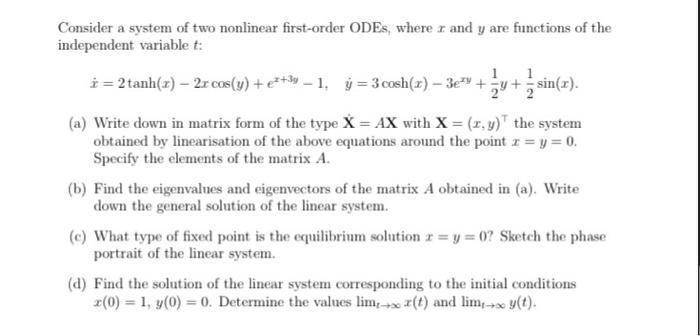Home / Expert Answers / Calculus / consider-a-system-of-two-nonlinear-first-order-odes-where-x-and-y-are-functions-of-the-pa859

# (Solved): Consider a system of two nonlinear first-order ODEs, where $$x$$ and $$y$$ are functions of the ...Consider a system of two nonlinear first-order ODEs, where $$x$$ and $$y$$ are functions of the independent variable $$t$$ : $\dot{x}=2 \tanh (x)-2 x \cos (y)+e^{x+3 y}-1, \quad \dot{y}=3 \cosh (x)-3 e^{x y}+\frac{1}{2} y+\frac{1}{2} \sin (x) .$ (a) Write down in matrix form of the type $$\mathbf{X}=A \mathbf{X}$$ with $$\mathbf{X}=(x, y)^{\top}$$ the system obtained by linearisation of the above equations around the point $$x=y=0$$. Specify the elements of the matrix $$A$$. (b) Find the eigenvalues and eigenvectors of the matrix $$A$$ obtained in (a). Write down the general solution of the linear system. (c) What type of fixed point is the equilibrium solution $$x=y=0$$ ? Sketch the phase portrait of the linear system. (d) Find the solution of the linear system corresponding to the initial conditions $$x(0)=1, y(0)=0$$. Determine the values $$\lim _{t \rightarrow \infty} x(t)$$ and $$\lim _{t \rightarrow \infty} y(t)$$.

We have an Answer from Expert